## Example Questions

### Example Question #1 : How To Find The Length Of A Radius

A circle has an area of 36π inches. What is the radius of the circle, in inches?

18

36

6

9

6

Explanation:

We know that the formula for the area of a circle is πr2. Therefore, we must set 36π equal to this formula to solve for the radius of the circle.

36π = πr2

36 = r2

6 = r

### Example Question #3 : How To Find The Length Of A Radius

Circle X is divided into 3 sections: A, B, and C. The 3 sections are equal in area. If the area of section C is 12π, what is the radius of the circle?Circle X

6

7

√12

4

6

Explanation:

Find the total area of the circle, then use the area formula to find the radius.

Area of section A = section B = section C

Area of circle X = A + B + C = 12π+ 12π + 12π = 36π

Area of circle =  where r is the radius of the circle

36π = πr2

36 = r2

√36 = r

6 = r

### Example Question #4 : How To Find The Length Of A Radius

The specifications of an official NBA basketball are that it must be 29.5 inches in circumference and weigh 22 ounces.  What is the approximate radius of the basketball?

9.39 inches

4.70 inches

3.06 inches

14.75 inches

5.43 inches

4.70 inches

Explanation:

To Find your answer, we would use the formula:  C=2πr. We are given that C = 29.5. Thus we can plug in to get  [29.5]=2πr and then multiply 2π to get 29.5=(6.28)r.  Lastly, we divide both sides by 6.28 to get 4.70=r.   (The information given of 22 ounces is useless)

### Example Question #8 : How To Find The Length Of A Radius

A circle with center (8, 5) is tangent to the y-axis in the standard (x,y) coordinate plane. What is the radius of this circle?

5

8

16

4

8

Explanation:

For the circle to be tangent to the y-axis, it must have its outer edge on the axis. The center is 8 units from the edge.

### Example Question #10 : How To Find The Length Of A Radius

A circle has an area of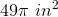. What is the radius of the circle, in inches?

49 inches

16 inches

24.5 inches

7 inches

14 inches

7 inches

Explanation:

We know that the formula for the area of a circle is πr2. Therefore, we must set 49π equal to this formula to solve for the radius of the circle.

49π = πr2

49 = r2

7 = r

### Example Question #591 : Geometry

A circle has a circumference of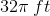. What is the radius of the circle, in feet?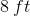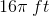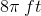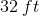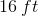Explanation:

To answer this question we need to find the radius of the circle given the circumference of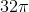.

The equation for a circle's circumference is: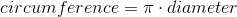We can plug our circumference into this equation to find the diameter.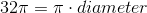We can now divide both sides by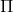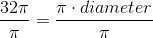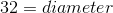So our diameter is. To find the radius from the diameter, we use the following equation: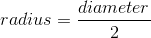So, for this data: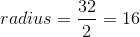Therefore, the radius of our circle is.